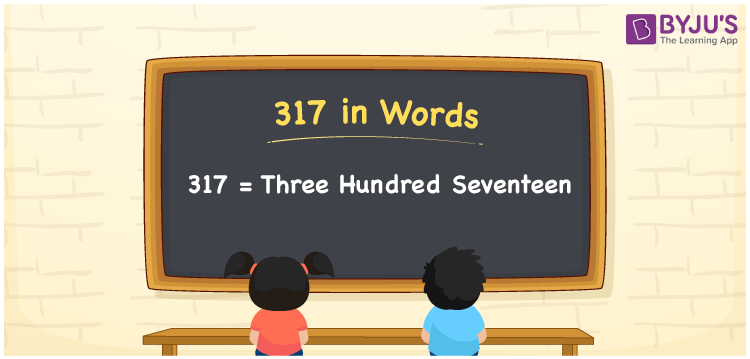# 317 in Words

317 in words is Three Hundred Seventeen. For example, if you buy a compact powder for Rs. 317, then you can say, “I bought a compact powder for Rupees Three Hundred Seventeen”. 317 is a cardinal number since it represents the value of something. Learning the concept of numbers in words and counting enhances the basics of Mathematics. Let us learn the conversion of the number 317 to words and its interesting facts in this article.

 317 in Words Three Hundred Seventeen Three Hundred Seventeen in numerical form 317

## 317 in English Words

English is the most important language used in education worldwide. Hence, we generally, write the numbers in words using the letters of the English alphabet. Therefore, we can read the number 317 in English as Three Hundred Seventeen.## How to Write 317 in Words?

317 is a three-digit number. Hence, we need a table of 3 columns to create the place value chart for the number 317 as shown in the below table.

 Hundreds Tens Ones 3 1 7

Therefore, we can write the expanded form as:

3 x Hundred + 1 x Ten + 7 x One

= 3 x 100 + 1 x 10 + 7 x 1

= 300 + 10 + 7

= 317

= Three Hundred Seventeen

Hence, 317 in words is written as Three Hundred Seventeen

Interesting way of writing 317 in words

3 = Three

31 = Thirty-One

317 = Three Hundred Seventeen

Thus, the word form of the number 317 is Three Hundred Seventeen

317 is a natural number that is the successor of 316 and the predecessor of 318

• 317 in words – Three Hundred Seventeen
• Is 317 an odd number? – Yes
• Is 317 an even number? – No
• Is 317 a perfect square number? – No
• Is 317 a perfect cube number? – No
• Is 317 a prime number? – Yes
• Is 317 a composite number? – No

## Frequently Asked Questions on 317 in Words

Q1

### How to write 317 in words?

317 in words can be written as Three Hundred Seventeen.
Q2

### Simplify 400 – 83, and express in words.

Simplifying 400 – 83, we get 317. Hence, the number 317 in words is Three Hundred Seventeen.
Q3

### Is 317 an odd number?

Yes, the number 317 is an odd number.